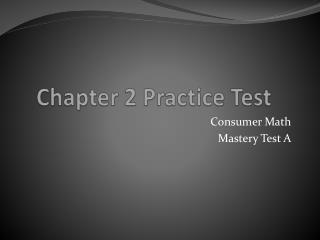DownloadDownload PresentationChapter 2 Practice Test

# Chapter 2 Practice Test

Télécharger la présentation## Chapter 2 Practice Test

- - - - - - - - - - - - - - - - - - - - - - - - - - - E N D - - - - - - - - - - - - - - - - - - - - - - - - - - -
##### Presentation Transcript

1. Chapter 2 Practice Test Consumer Math Mastery Test A

2. 465¢ 72¢ 12¢ \$0.53 \$1.95 \$0.03

3. 7. How much is one package of corn? \$1.89 ÷ 2 = 0.945, round, \$0.95 8. Mrs. Valle buys 10 pounds of potatoes, two apple pies, and one can of peaches. How much is her total bill? \$2.79 + \$3.85 + \$3.85 + \$1.00 = \$11.49 9. If Mrs. Valle gives the salesclerk \$20, how much is her change? \$20.00 - \$11.49 = \$8.51

4. 10. Find the unit price of Smith’s Popcorn. How much does it cost per ounce? \$1.28 ÷ 15 = 0.0853333, round, 9¢ 11. Find the unit price of Topper Popcorn. How much does it cost per ounce? \$1.42 ÷ 32 = 0.044375, round, 4¢ 12. Oscar uses a 20¢ off coupon when he buys Super Window Cleaner. How much does this window cleaner cost per ounce? \$1.80 - \$0.20 = \$1.60, \$1.60 ÷ 17 = 0.0941176, round, 9¢

5. 13. Tanya and Nerissa eat dinner at a restaurant. The bill totals \$23.41. They want to leave a 15% tip. How much should each person pay? • \$23.41 x .15 = \$3.5115 • Round to \$3.51 • \$23.41 + \$3.51 = \$26.92 • \$26.92 ÷ 2 = \$13.46

6. D; 3¢ A; 7¢